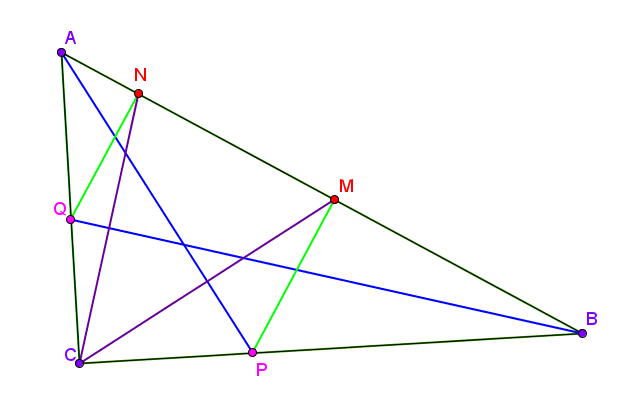# What name shall I give to this problem?

Geometry Level 3Let $\Delta ABC$ be a right angled triangle with $\angle C = 90^{o}$. Suppose that $AP$ and $BQ$ be the angular bisectors of $\angle CAB$ and $\angle CBA$ respectively. Let $M$ and $N$ be perpendiculars respectively from $P$ and $Q$ on to $AB$. Find the value of $\angle MCN$ (in degree).

###### This problem is from the set What name should I give?
×

Problem Loading...

Note Loading...

Set Loading...